# R S Aggarwal Solutions for Class 11 Maths Chapter 28 Differentiation Exercise 28B

Exercise 28B of Chapter 28 – Differentiation, problems are based on “derivative from the first principle”. All questions are solved by subject experts at BYJU’S with step-by-step explanation which helps students in understanding the concepts easily and quickly. Download R S Aggarwal Class 11 Chapter 28 exercise 28B solutions from the link given below.

Class 11 Maths Chapter 28 Differentiation Exercise 28B is based on the topic: Derivative from the first principle.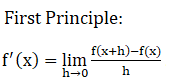## Download PDF of R S Aggarwal Solutions for Class 11 Maths Chapter 28 Differentiation Exercise 28B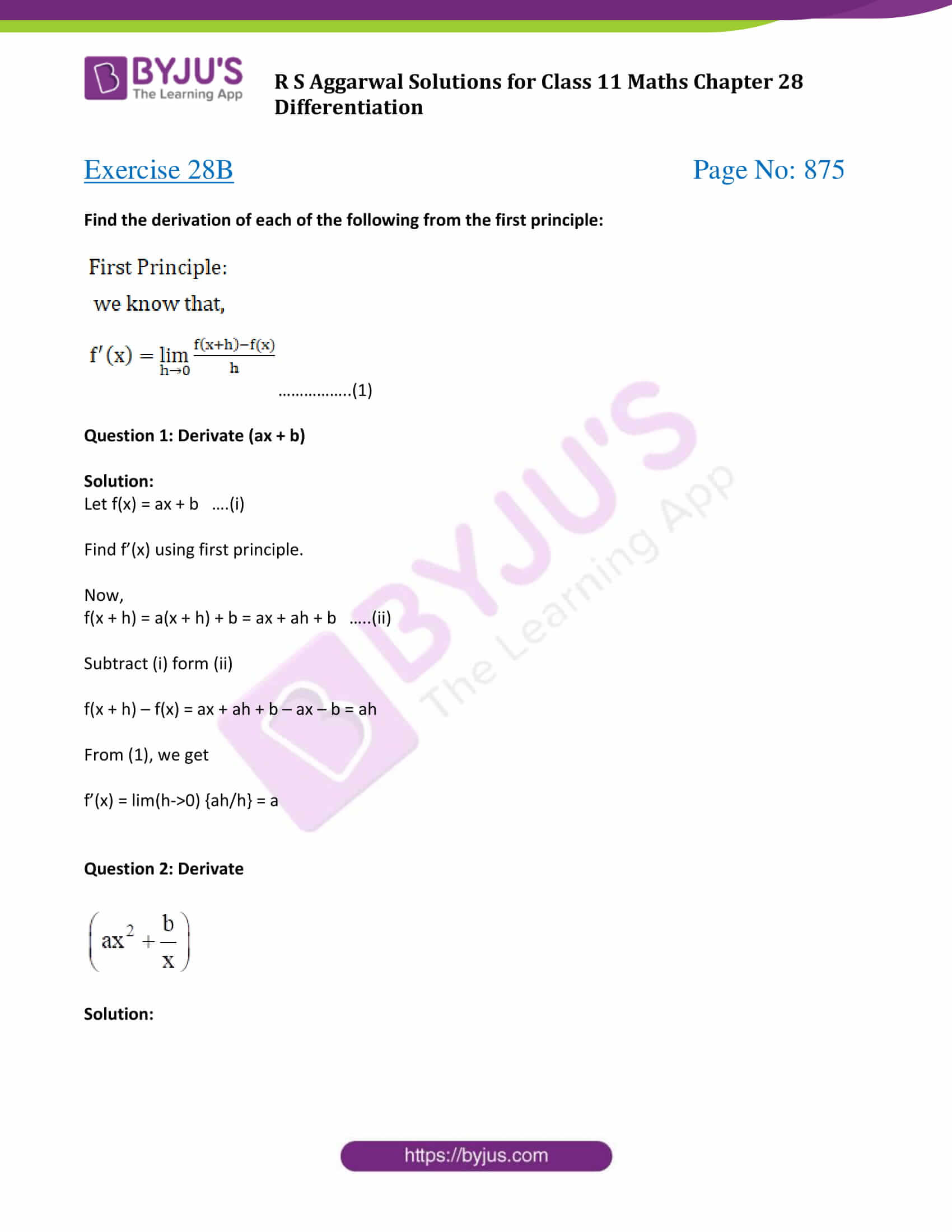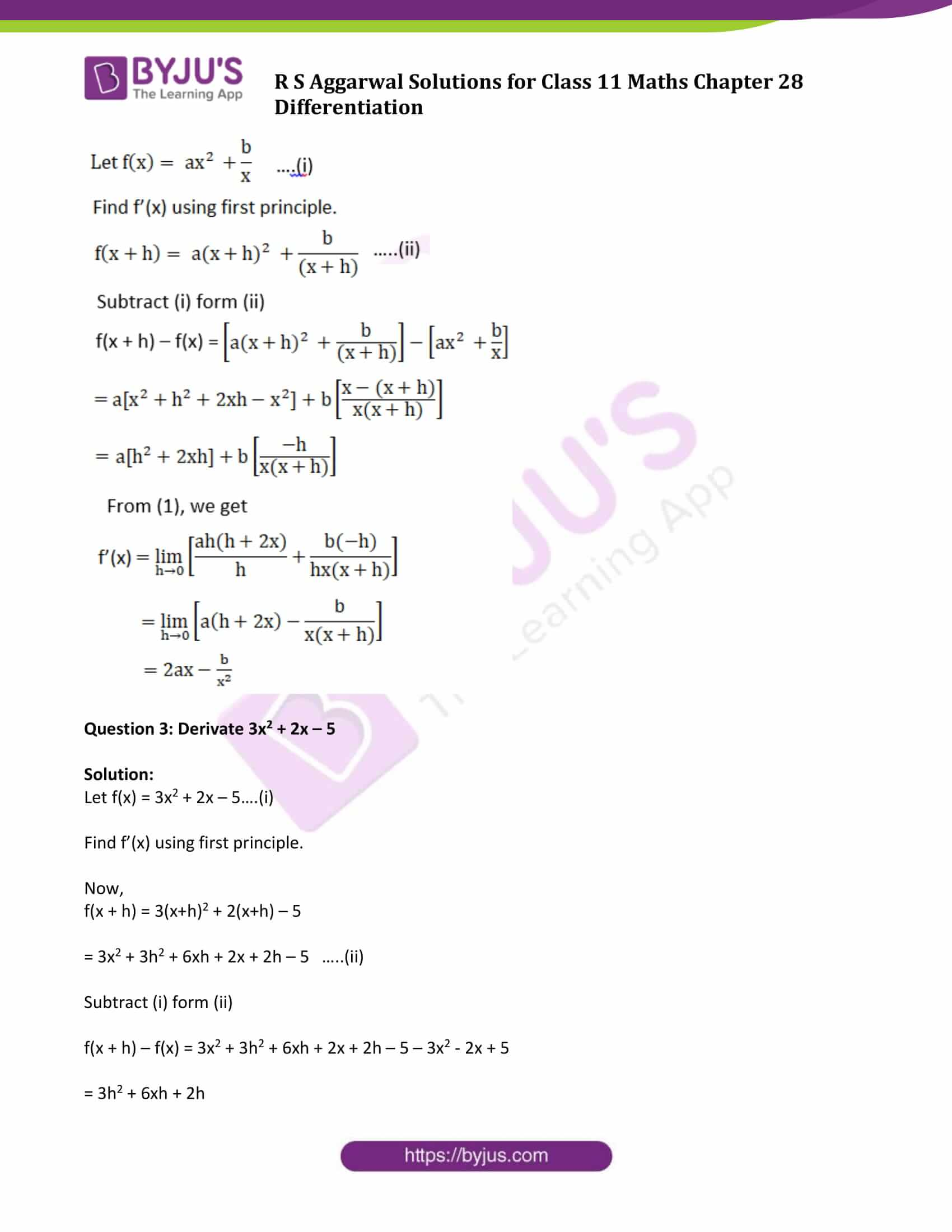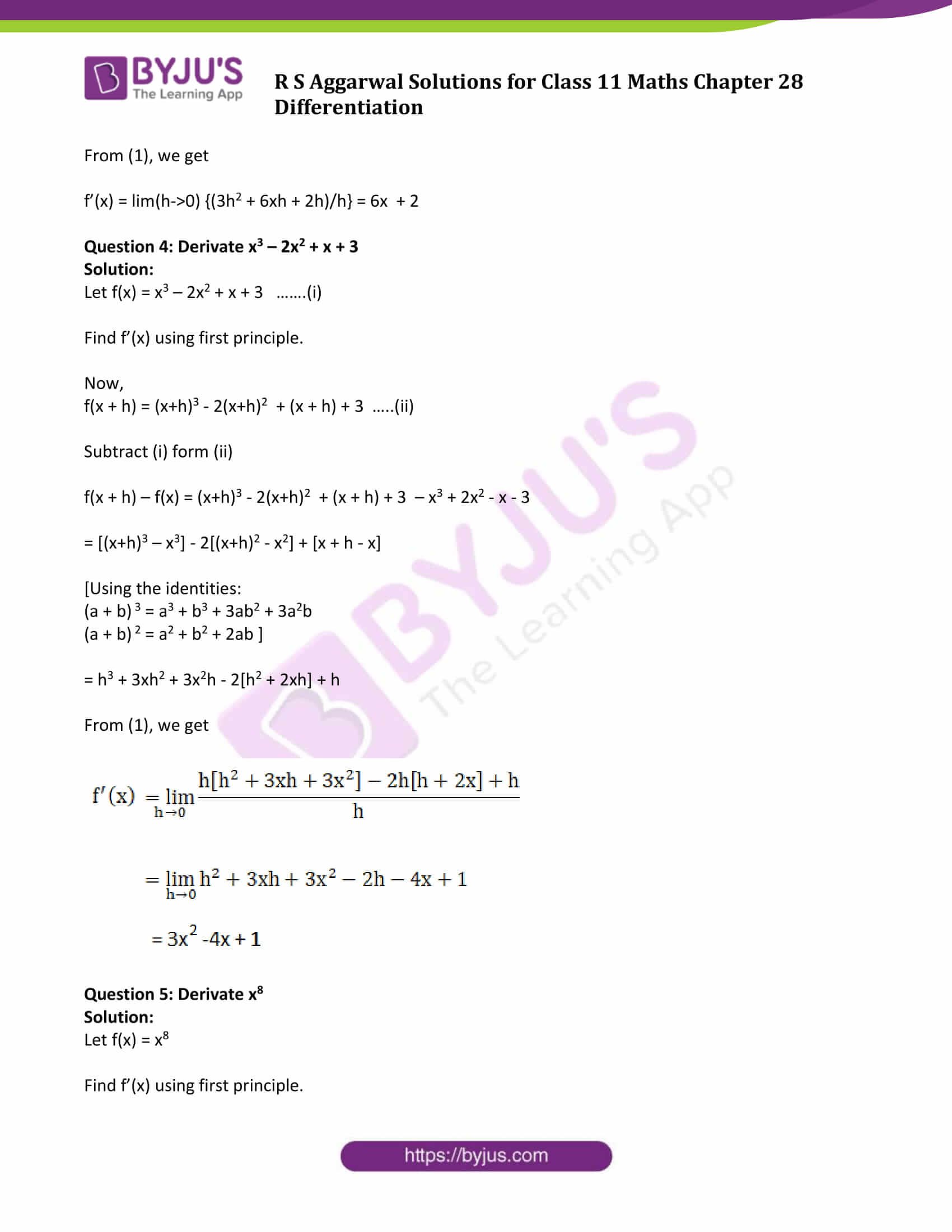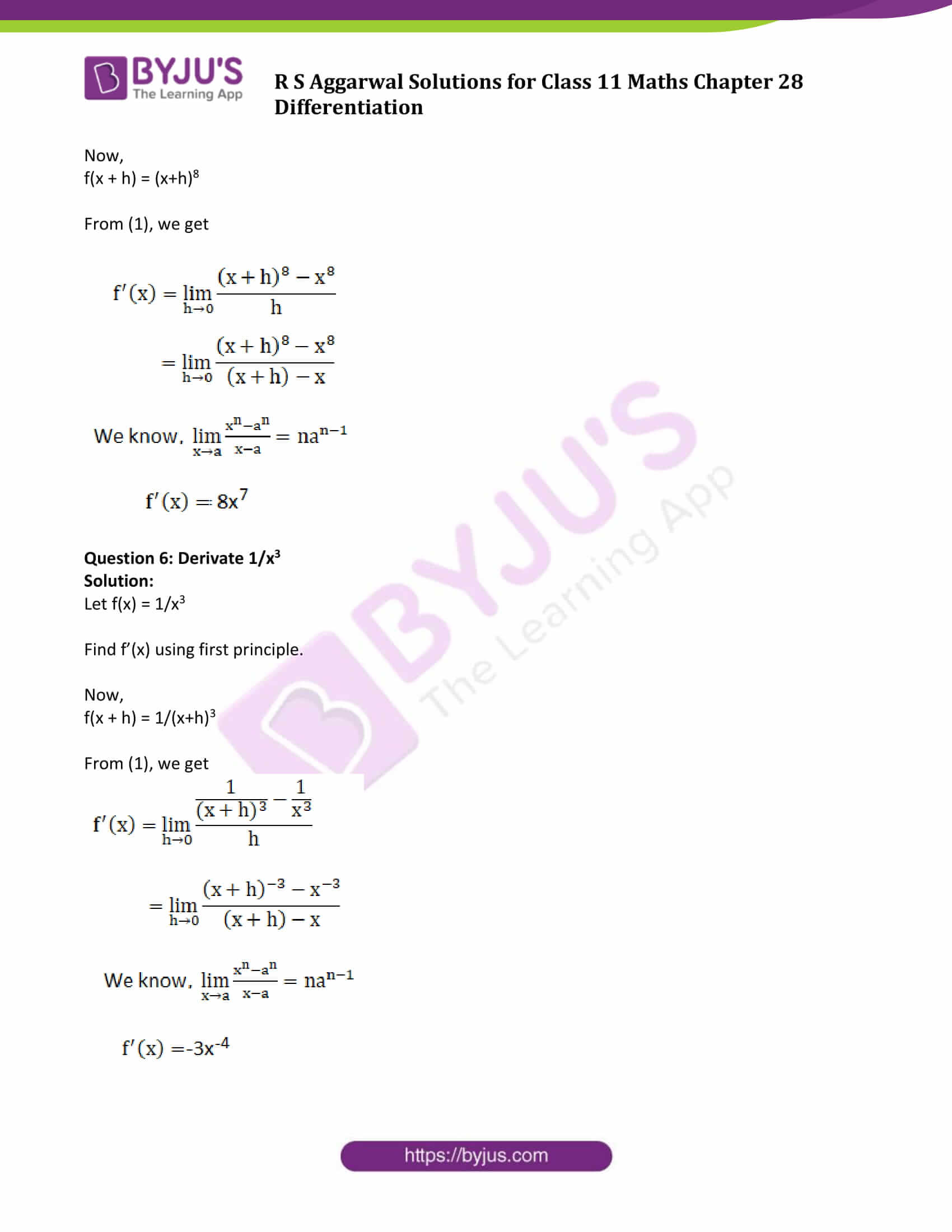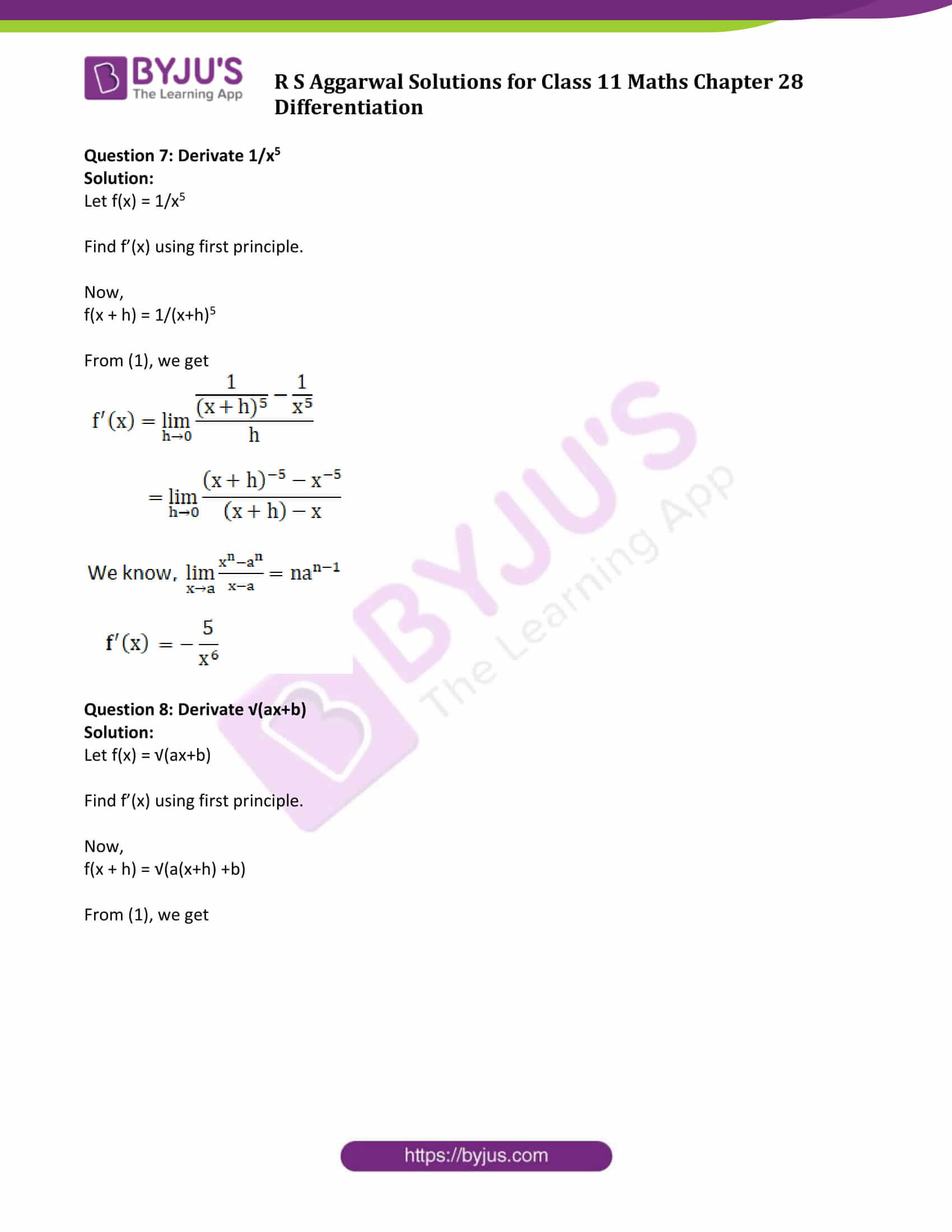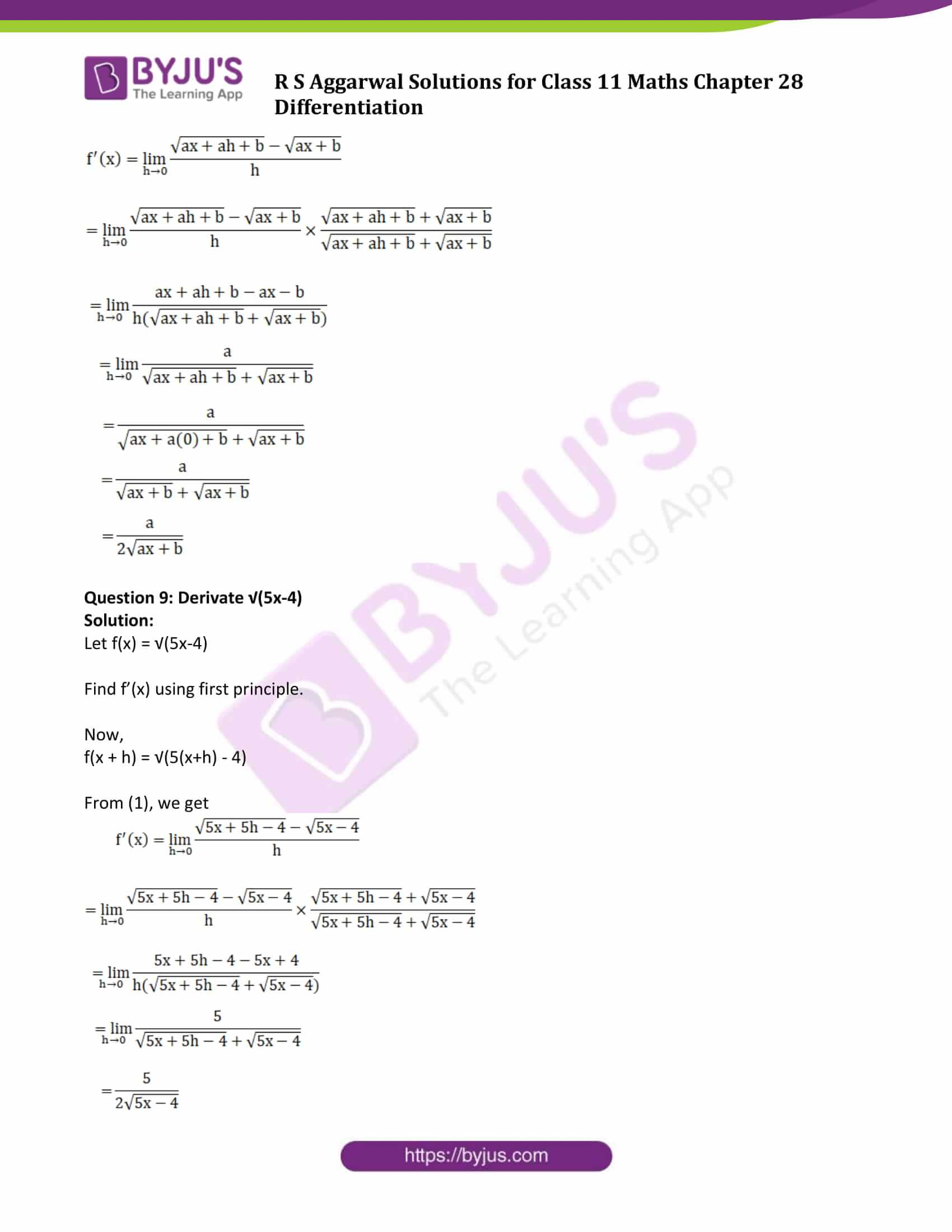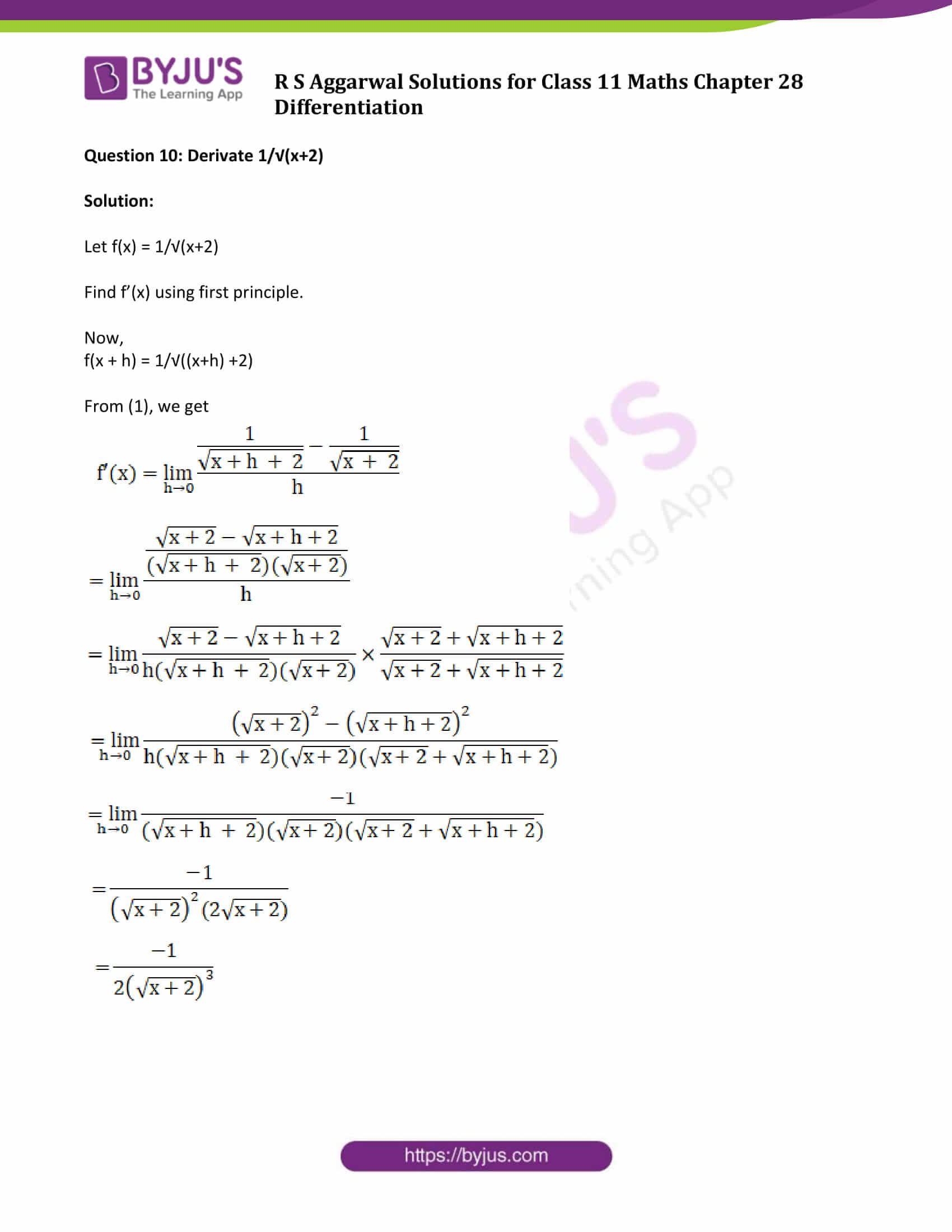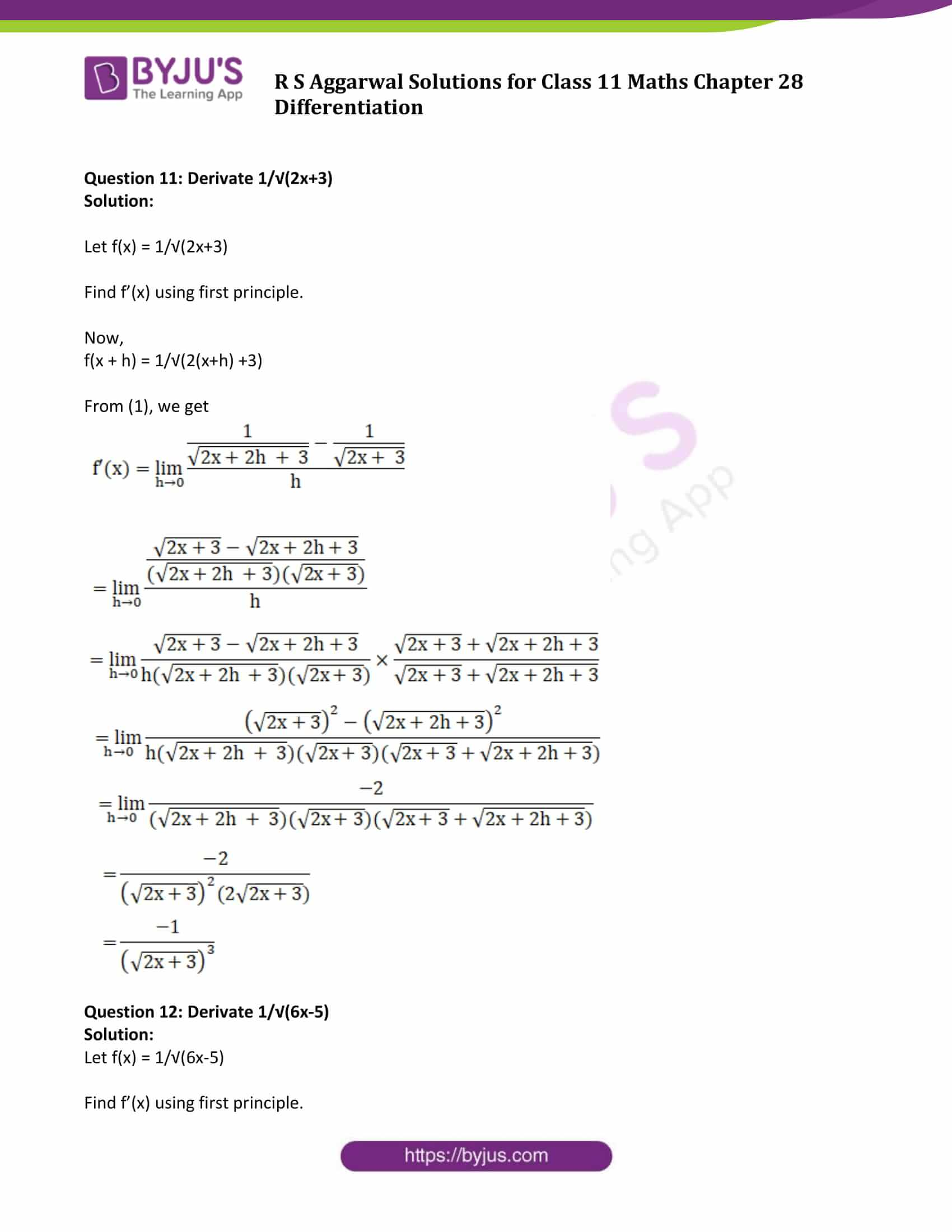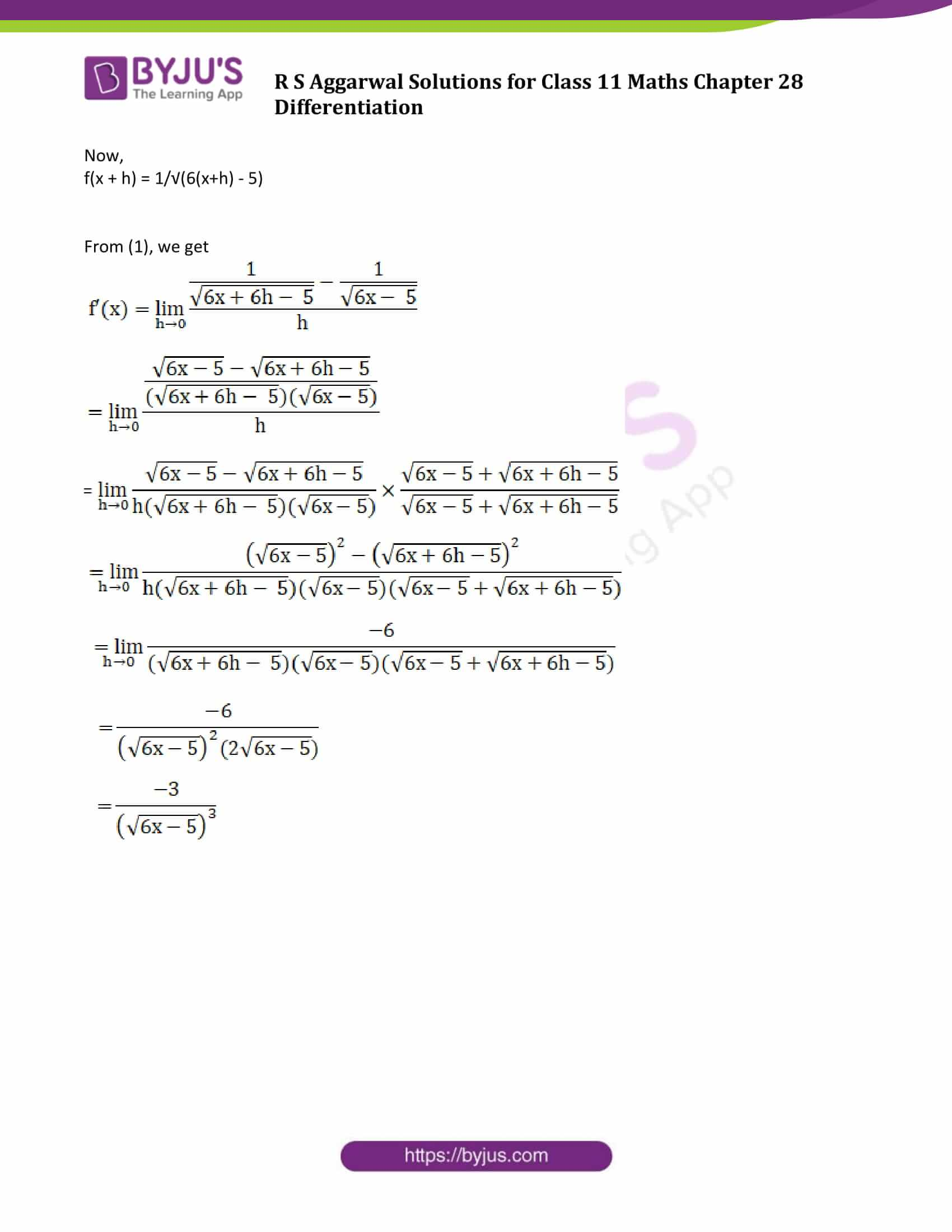### Access Answers to Maths R S Aggarwal Class 11 Chapter 28 Differentiation Exercise 28B Page number 875

Find the derivation of each of the following from the first principle: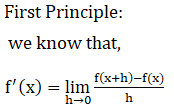……………..(1)

Question 1: Derivate (ax + b)

Solution:

Let f(x) = ax + b ….(i)

Find f’(x) using first principle.

Now,

f(x + h) = a(x + h) + b = ax + ah + b …..(ii)

Subtract (i) form (ii)

f(x + h) – f(x) = ax + ah + b – ax – b = ah

From (1), we get

f’(x) = lim(h->0) {ah/h} = a

Question 2: Derivate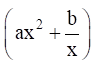Solution: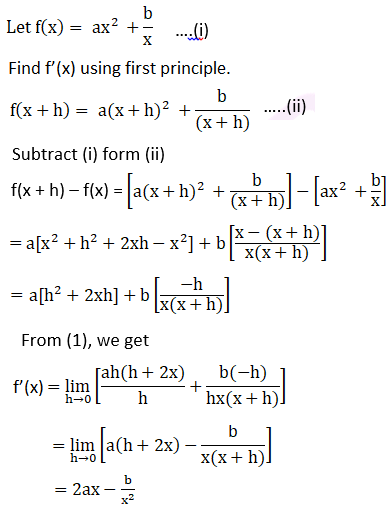Question 3: Derivate 3x2 + 2x – 5

Solution:

Let f(x) = 3x2 + 2x – 5….(i)

Find f’(x) using first principle.

Now,

f(x + h) = 3(x+h)2 + 2(x+h) – 5

= 3x2 + 3h2 + 6xh + 2x + 2h – 5 …..(ii)

Subtract (i) form (ii)

f(x + h) – f(x) = 3x2 + 3h2 + 6xh + 2x + 2h – 5 – 3x2 – 2x + 5

= 3h2 + 6xh + 2h

From (1), we get

f’(x) = lim(h->0) {(3h2 + 6xh + 2h)/h} = 6x + 2

Question 4: Derivate x3 – 2x2 + x + 3

Solution:

Let f(x) = x3 – 2x2 + x + 3 …….(i)

Find f’(x) using first principle.

Now,

f(x + h) = (x+h)3 – 2(x+h)2 + (x + h) + 3 …..(ii)

Subtract (i) form (ii)

f(x + h) – f(x) = (x+h)3 – 2(x+h)2 + (x + h) + 3 – x3 + 2x2 – x – 3

= [(x+h)3 – x3] – 2[(x+h)2 – x2] + [x + h – x] [Using the identities:

(a + b) 3 = a3 + b3 + 3ab2 + 3a2b

(a + b) 2 = a2 + b2 + 2ab ]

= h3 + 3xh2 + 3x2h – 2[h2 + 2xh] + h

From (1), we get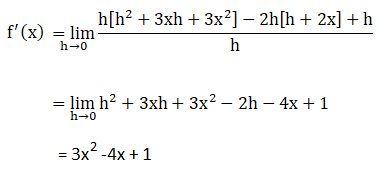Question 5: Derivate x8

Solution:

Let f(x) = x8

Find f’(x) using first principle.

Now,

f(x + h) = (x+h)8

From (1), we get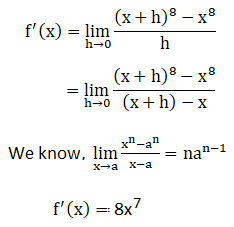Question 6: Derivate 1/x3

Solution:

Let f(x) = 1/x3

Find f’(x) using first principle.

Now,

f(x + h) = 1/(x+h)3

From (1), we get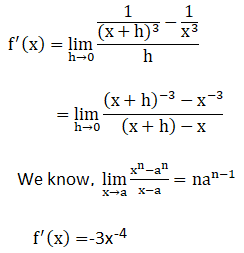Question 7: Derivate 1/x5

Solution:

Let f(x) = 1/x5

Find f’(x) using first principle.

Now,

f(x + h) = 1/(x+h)5

From (1), we get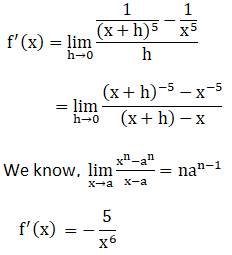Question 8: Derivate √(ax+b)

Solution:

Let f(x) = √(ax+b)

Find f’(x) using first principle.

Now,

f(x + h) = √(a(x+h) +b)

From (1), we get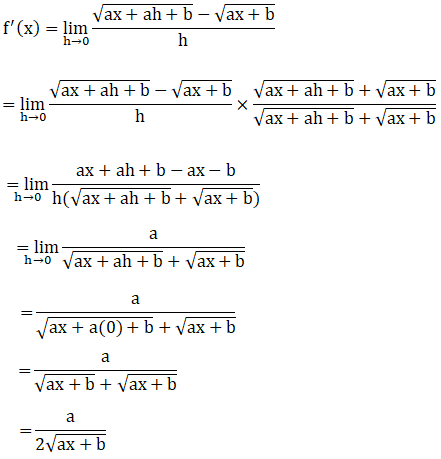Question 9: Derivate √(5x-4)

Solution:

Let f(x) = √(5x-4)

Find f’(x) using first principle.

Now,

f(x + h) = √(5(x+h) – 4)

From (1), we get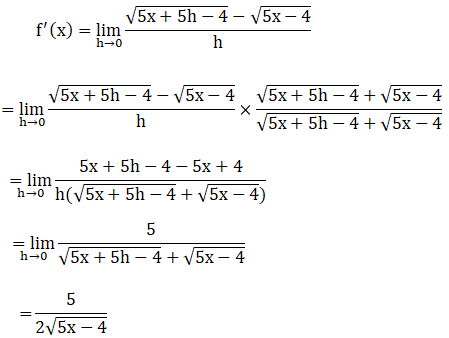Question 10: Derivate 1/√(x+2)

Solution:

Let f(x) = 1/√(x+2)

Find f’(x) using first principle.

Now,

f(x + h) = 1/√((x+h) +2)

From (1), we get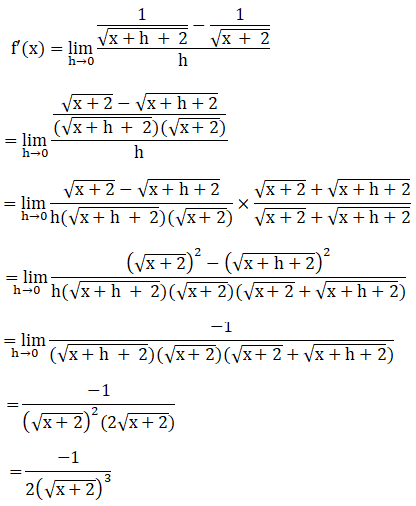Question 11: Derivate 1/√(2x+3)

Solution:

Let f(x) = 1/√(2x+3)

Find f’(x) using first principle.

Now,

f(x + h) = 1/√(2(x+h) +3)

From (1), we get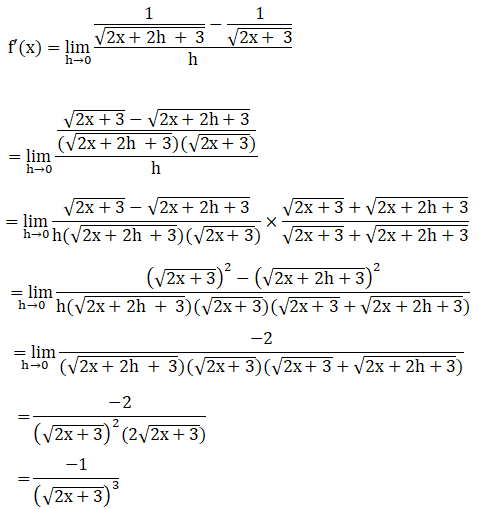Question 12: Derivate 1/√(6x-5)

Solution:

Let f(x) = 1/√(6x-5)

Find f’(x) using first principle.

Now,

f(x + h) = 1/√(6(x+h) – 5)

From (1), we get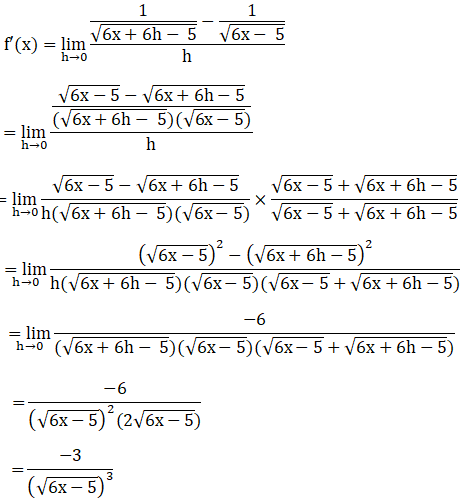## Access other exercise solutions of Class 11 Maths Chapter 28 Differentiation

Exercise 28A Solutions

Exercise 28C Solutions

Exercise 28D Solutions

Exercise 28E Solutions